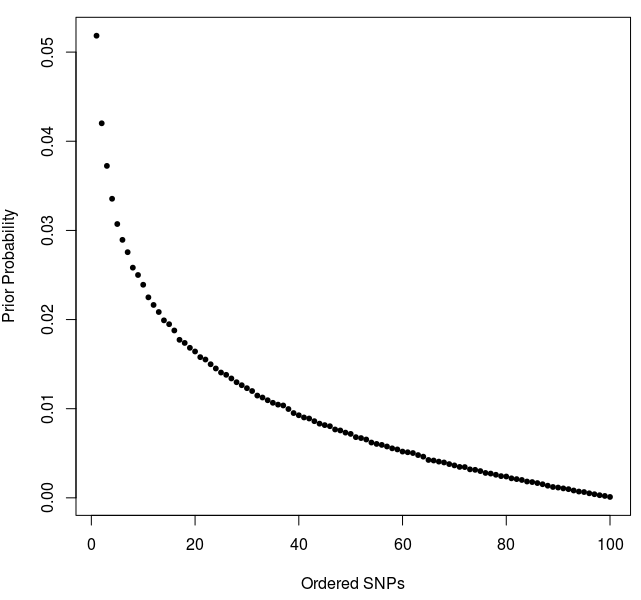### 1. Assessing empirical coverage

I investigate whether the top PP has accurate empirical coverage when calculated using the standard method (original PPs) and the reweighting method (reweighted PPs). To do this, I store the maximum PP value and a binary indicator of whether this SNP is the causal SNP. I repeat this many times using a variety of parameters to vary the power of the simulations.

Note, the prior for the reweighting method was generated by:

``prior <- rowMeans(sapply(1:1000000, function(i) draw_order(nsnps = 100, 1)))``The following results are for 25,000 low LD region simulations and 25,000 high LD region simulations, whereby each data point is the average of ~1500 simulations for a specified PP bin (\(x\) is the mean PP value in the interval and \(y\) is the mean binary `is.CV` value).